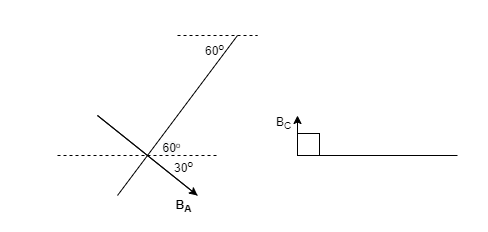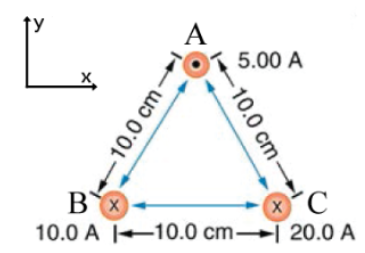# Problem: The figure shows a cross-section of three long straight current-carrying wires. Notice we are using the common convention that crosses denote vectors going into the page and dots denote vectors coming out of the page Part (a) Find the magnitude of the force per units length, in newtons per meter, on wire B.Part (b) Find the direction of the force for wire B, in degrees. Measure this angle counterclockwise, in degrees, from the positive x-axis.

###### FREE Expert Solution

Magnetic field due to straight currents:

$\overline{){\mathbf{B}}{\mathbf{=}}\frac{{\mathbf{\mu }}_{\mathbf{0}}\mathbf{i}}{\mathbf{2}\mathbf{\pi }\mathbf{r}}}$

Magnetic force on a current-carrying wire:

$\overline{){\mathbf{F}}{\mathbf{=}}{\mathbf{B}}{\mathbf{i}}{\mathbf{L}}{\mathbf{s}}{\mathbf{i}}{\mathbf{n}}{\mathbf{\theta }}}$

(a)

We'll determine the magnetic force at B due to wires A and C. We'll then use the resultant magnetic field to determine the force on B.

The magnetic field around conductors is represented by concentric circles.

The direction at a point is given by the tangent at that point.

Right-hand rule: Thumb - current. Finger curl in direction of the magnetic field.Using Right-hand rule, the magnetic field due to conductor C is directed in a clockwise direction. The field due to conductor A is directed in a counterclockwise direction.

91% (117 ratings)###### Problem Details

The figure shows a cross-section of three long straight current-carrying wires. Notice we are using the common convention that crosses denote vectors going into the page and dots denote vectors coming out of the pagePart (a) Find the magnitude of the force per units length, in newtons per meter, on wire B.

Part (b) Find the direction of the force for wire B, in degrees. Measure this angle counterclockwise, in degrees, from the positive x-axis.